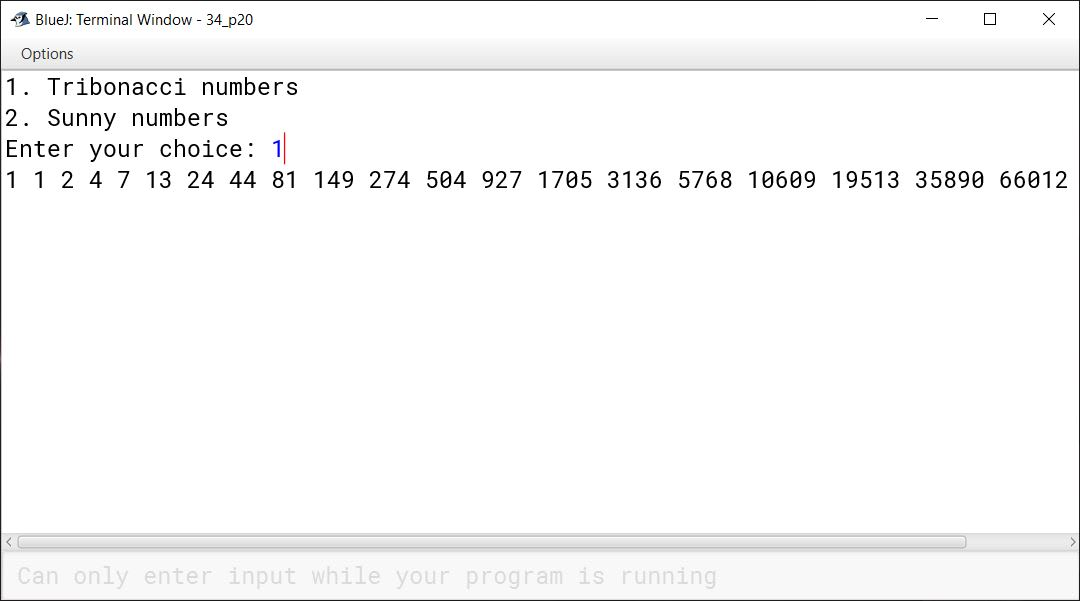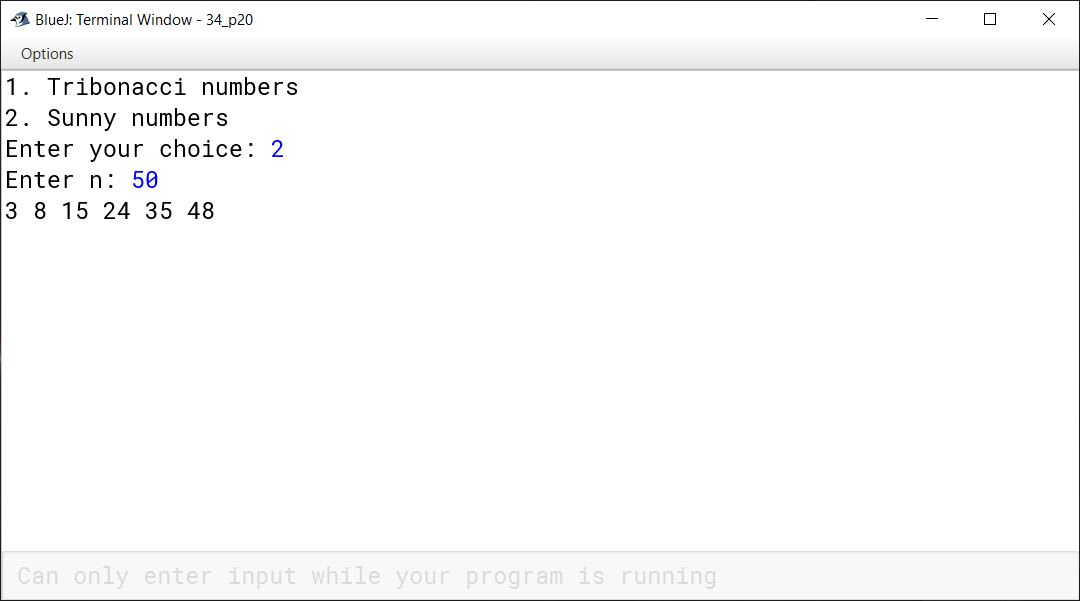# Iterative Constructs in Java

## Multiple Choice Questions

#### Question 1

Which of the following statements will terminate the premature execution of a program?

1. System.exit(0)
2. break
3. stop
4. end

System.exit(0)

Reason — As soon as System.exit(0) function is invoked, it terminates the execution, ignoring the rest of the statements of the program.

#### Question 2

Which of the following for loop will not be an infinite loop?

1. for(; ;)
2. for(a = 0; a < 1; a--)
3. for(a = 0; ; a++)
4. for(a = -1; a < 1; a++)

for(a = -1; a < 1; a++)

Reason — In the selected for loop, the initial value of a (-1) will be incremented by 1 every time and eventually the condition (a < 1) will become false and the loop will terminate.

#### Question 3

Choose the odd one out from the following:

1. return
2. break
3. continue
4. if-else

if-else

Reason — if-else is a conditional statement while return, break and continue are jump statements.

#### Question 4

The while statement repeats the execution of a block only when the given condition is:

1. False
2. True
3. 1
4. 0

True

Reason — The while statement repeats the execution of a block only when the given condition is true.

#### Question 5

Which of the following is not a part of the looping construct?

1. close
2. for
3. break
4. continue

close

Reason — Close is not a part of the looping construct.

## Fill in the blanks

#### Question 1

When the statements are repeated sequentially a number of times in a program, the construct is known as loop.

#### Question 2

For loop is also known as entry controlled loop.

#### Question 3

do-while loop is called an exit controlled loop.

#### Question 4

do-while loop executes at least once, if the condition is false.

#### Question 5

while loop checks the condition first before its execution.

#### Question 6

To find the sum of any ten numbers, the loop will run ten times.

## Predict the output

#### Question 1

The following is a segment of a program.

```x = 1; y = 1;
if(n > 0)
{
x = x + 1;
y = y + 1;
}```

What will be the value of x and y, if n assumes a value:

(a) 1      (b) 0

(a) When n = 1

##### Output
``````x = 2
y = 2
``````
##### Explanation

As `n` is 1, so if condition is `true`. `x` and `y` are incremented by 1 so both become 2.

(a) When n = 0

##### Output
``````x = 1
y = 1
``````
##### Explanation

As `n` is 1, so if condition is `false`. Values of both `x` and `y` remain unchanged.

#### Question 2

Analyze the following program segment and determine how many times the body of the loop will be executed (show the working).

```x = 5; y = 50;
while(x <= y)
{
y = y / x;
System.out.println(y);
}```

##### Output
``````10
2
``````

The loop will execute 2 times.

##### Explanation
xyRemarks
550Initial values
510After 1st iteration
52After 2nd iteration

After 2 iterations y becomes less than x so condition of while loop becomes false and it stops executing.

#### Question 3

What will be the output of the following code?

```int m = 2;
int n = 15;
for(int i = 1 ; i < 5 ; i++)
m++;
--n;
System.out.println(" m = " + m);
System.out.println(" n = " + n);```

##### Output
``````m = 6
n = 14
``````
##### Explanation

As there are no curly braces after the for loop so only the statement `m++;` is inside the loop. Loop executes 4 times so `m` becomes 6. The next statement `--n;` is outside the loop so it is executed only once and `n` becomes 14.

#### Question 4

Analyze the following program segment and determine how many times the loop will be executed. What will be the output of the program segment?

```int k = 1, i = 2;
while(++i < 6)
k *= i;
System.out.println(k);```

##### Output
``````60
``````
##### Explanation

This table shows the change in values of i and k as while loop iterates:

ikRemarks
21Initial values
331st Iteration
4122nd Iteration
5603rd Iteration
660Once i becomes 6, condition is false and loop stops iterating.

Notice that `System.out.println(k);` is not inside while loop. As there are no curly braces so only the statement `k *= i;` is inside the loop. The statement `System.out.println(k);` is outside the while loop, it is executed once and prints value of k which is 60 to the console.

#### Question 5

Give the output of the following program segment and also mention the number of times the loop is executed.

```int a , b;
for(a = 6 ; b = 4; a <= 4; a = a + 6)
{
if(a % b == 0)
break;
}
System.out.println(a);```

##### Output
``````6
``````

The loop executes 0 times.

##### Explanation

`a` is initialized to 6 and as the loop condition is false before the first iteration itself so the loop doesn't execute.

#### Question 6

Give the output of the following program segment and also mention how many times the loop is executed.

```int i;
for(i = 5 ; i > 10 ; i++)
System.out.println(i);
System.out.println(i * 4);```

##### Output
``````20
``````

The loop executes 0 times.

##### Explanation

`i` is initialized to 5 and as the loop condition is false before the first iteration itself so the loop doesn't execute. The statement `System.out.println(i * 4);` is outside the loop so it gets executed once, printing 20 to the console.

## Rewrite the following programs

#### Question 1

Using for loop:

```int i = 1;
int d = 5;
do
{
d = d * 2;
System.out.println(d);
i++;
}
while (i <= 5);```

```int d = 5;
for (int i = 1 ; i <= 5 ; i++) {
d = d * 2;
System.out.println(d);
}```

#### Question 2

Using while loop:

```import java.util.*;
class Number
{
public static void main(String args[])
{
int n , r;
Scanner in = new Scanner(System.in);
System.out.println("Enter a number");
n = in.nextInt();
do
{
r = n % 10;
n = n / 10;
System.out.println(r);
}
while(n != 0);
}
}```

```import java.util.*;
class Number
{
public static void main(String args[])
{
int n , r;
Scanner in = new Scanner(System.in);
System.out.println("Enter a number");
n = in.nextInt();
while (n != 0) {
r = n % 10;
n = n / 10;
System.out.println(r);
}
}
}```

#### Question 1

What is for loop? What are the parameters used in for loop?

The for loop is used when number of iterations is fixed and known. It is also referred to as a fixed or known iterative looping construct.

The following parameters are commonly used in a for loop:

1. An initial value for the loop control variable.
2. A condition—loop will iterate as long as this condition remains true.
3. An update expression to modify the loop control variable after every iteration.
4. Body of the loop which consists of the statements that needs to be repeatedly executed.

Below is an example of for loop, it prints the table of 2 till 12:

```for (int i = 1 ; i <= 12 ; i++) {
int a = 2 * i;
System.out.println("2 x " + i + "\t= " + a);
}```

#### Question 2

Define the following with their constructs:

(a) Entry controlled loop

(b) Exit controlled loop

(a) Entry controlled loop — An entry-controlled loop checks the condition at the time of entry. Only if the condition is true, the program control enters the body of the loop. for and while loops are entry-controlled loops.

(b) Exit controlled loop — An exit-controlled loop checks the condition after executing its body. If the condition is true, loop will perform the next iteration otherwise program control will move out of the loop. do-while loop is an exit-controlled loop.

#### Question 3

Write down the syntax of:

(a) do - while

(b) while loop

(a) Syntax of do - while

``````do {
//loop-body
} while (condition);
``````

(b) Syntax of while loop

``````while (condition) {
//loop-body
}
``````

#### Question 4

What is the purpose of using:

(a) break statement

(b) continue statement

in a program

(a) break statement — break statement is used when the user would like to terminate the loop before its completion and send the control to exit the loop, if certain condition is true.

(b) continue statement — When continue statement is invoked, the control goes back to check the condition of the loop by ignoring rest of the statements of the loop.

#### Question 5

Distinguish between while and do-while loop.

whiledo-while
It is an entry-controlled loop.It is an exit-controlled loop.
It is helpful in situations where number of iterations are not known.It is suitable when we need to display a menu to the user.

#### Question 6

What is meant by an infinite loop? Give an example.

A loop that repeats for infinite number of iterations is known as infinite loop or endless loop.

Consider the example given below. Since all the parameters are missing in for loop, it will repeat for infinite number of times.

```for ( ; ; )
System.out.println("Infinite Loop");```

#### Question 7

State one difference and one similarity between while loop and do-while loop.

Similarity — Both while and do-while are suitable in situations where numbers of iterations is not known.

Difference — while is an entry-controlled loop whereas do-while is an exit-controlled loop.

## Solutions to Unsolved Java Programs

#### Question 1

Write the programs in Java to display the first ten terms of the following series:

(a) 0, 1, 2, 3, 6,

```public class KboatTribonacci
{
public void displaySeries() {

int a = 0, b = 1, c = 2;

System.out.print(a + ", " + b + ", " + c);

for (int i = 0; i < 7; i++) {
int n = a + b + c;
System.out.print(", " + n);
a = b;
b = c;
c = n;
}
}
}```
##### Output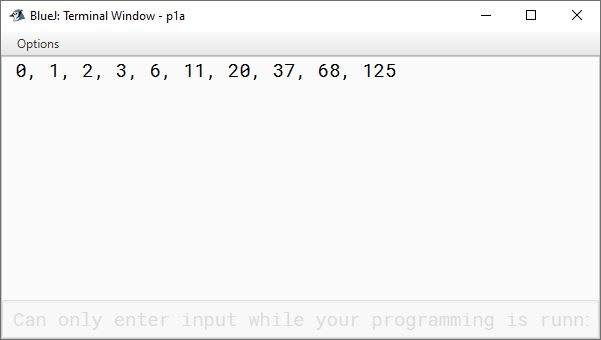(b) 1, -3, 5, -7, 9,

```public class KboatSeriesB
{
public void displaySeries() {

int term = 1;
System.out.print(term);

for (int i = 2; i <= 10; i++) {

term += 2;

if (i % 2 == 0)
System.out.print(", " + (-term));
else
System.out.print(", " + term);
}
}
}```
##### Output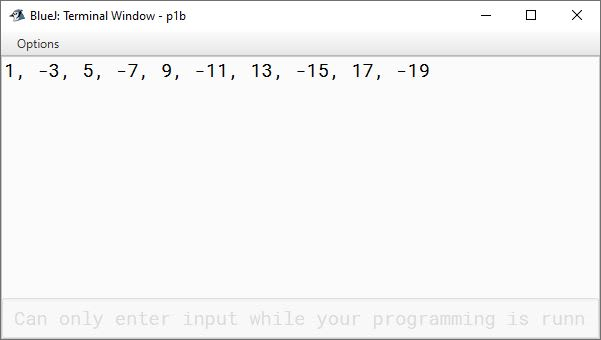(c) 0, 3, 8, 15,

```public class KboatSeries
{
public void generateSeries() {

int term = 0;
System.out.print(term);
for (int i = 3; i < 20; i = i + 2) {
term += i;
System.out.print(", " + term);
}
}
}```
##### Output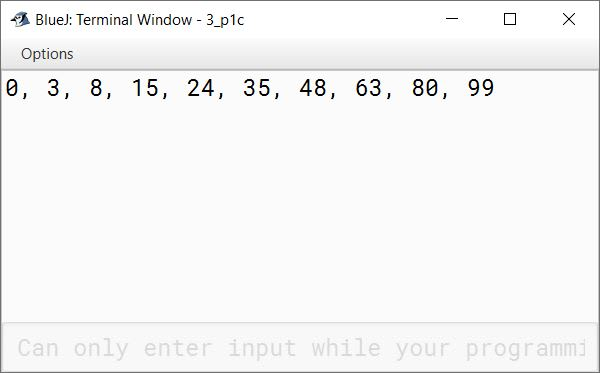(d) 1, 11, 111, 1111,

```public class KboatSeries
{
public void generateSeries() {
int term = 1;

for (int i = 1; i <= 10; i++) {
System.out.print(term + ", ");
term = term * 10 + 1;
}
}
}```
##### Output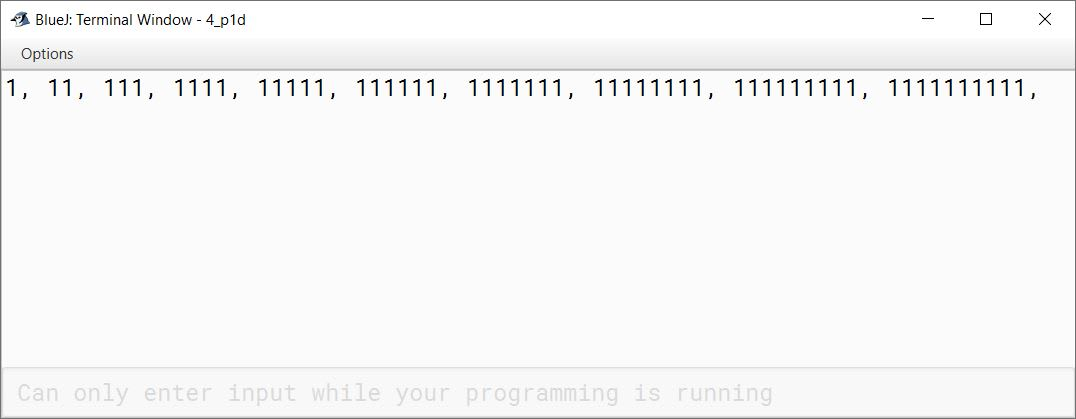(e) 1, 12, 123, 1234,

```public class KboatSeries
{
public void generateSeries() {

for (int i = 1; i <= 10; i++) {
for (int j = 1; j <= i; j++) {
System.out.print(j);
}
System.out.print(", ");
}

}
}```
##### Output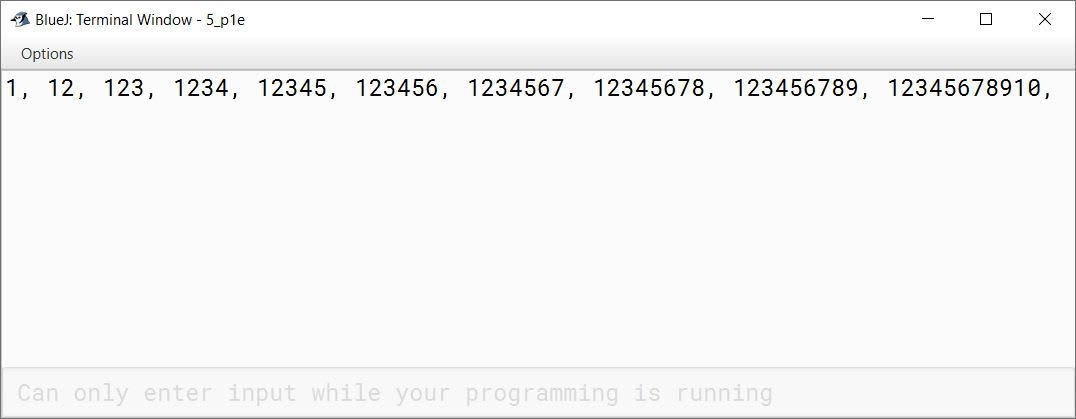#### Question 2

Write the programs in Java to find the sum of the following series:

(a) S = 1 + 1 + 2 + 3 + 5 + ....... to n terms

```import java.util.Scanner;

public class KboatSeries
{
public void computeSeriesSum() {

Scanner in = new Scanner(System.in);
System.out.print("Enter n: ");
int n = in.nextInt();

int a = 1, b = 1;
int sum = a + b;

for (int i = 3; i <= n; i++) {
int term = a + b;
sum += term;
a = b;
b = term;
}

System.out.println("Sum=" + sum);
}

}```
##### Output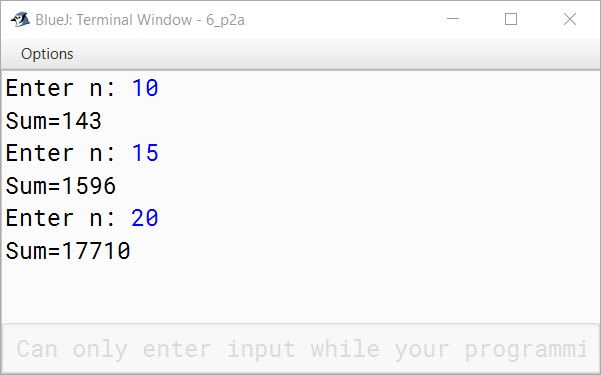(b) S = 2 - 4 + 6 - 8 + ....... to n

```import java.util.Scanner;

public class KboatSeries
{
public void computeSeriesSum() {

Scanner in = new Scanner(System.in);
System.out.print("Enter n: ");
int n = in.nextInt();

int sum = 0;

for (int i = 1, j = 2; i <= n; i++, j = j + 2) {
if (i % 2 == 0) {
sum -= j;
}
else {
sum += j;
}
}

System.out.println("Sum=" + sum);
}
}```
##### Output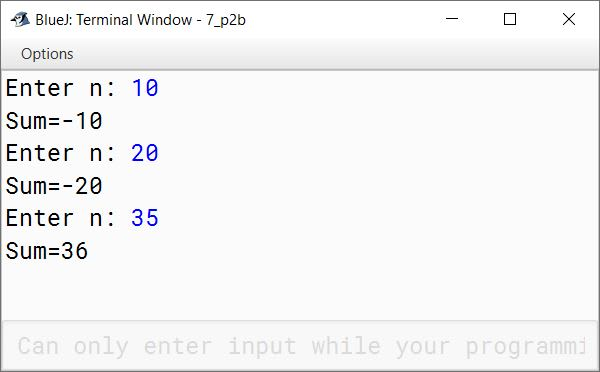(c) S = 1 + (1+2) + (1+2+3) + ....... + (1+2+3+ ....... + n)

```import java.util.Scanner;

public class KboatSeries
{
public void computeSeriesSum() {

Scanner in = new Scanner(System.in);
System.out.print("Enter n: ");
int n = in.nextInt();
int sum = 0, term = 0;

for (int i = 1; i <= n; i++) {
term += i;
sum += term;
}

System.out.println("Sum=" + sum);
}
}```
##### Output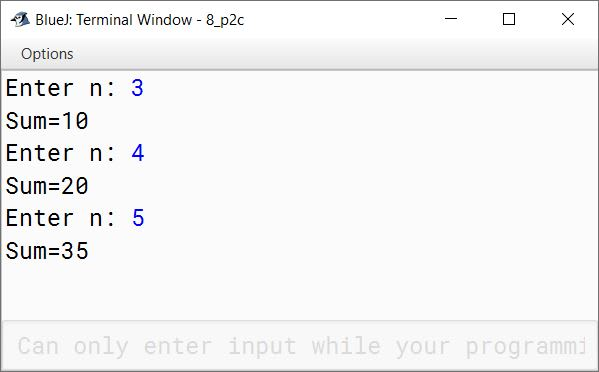(d) S = 1 + (1*2) + (1*2*3) + ....... + (1*2*3* ....... * n)

```import java.util.Scanner;

public class KboatSeries
{
public void computeSeriesSum() {

Scanner in = new Scanner(System.in);
System.out.print("Enter n: ");
int n = in.nextInt();
long sum = 0, term = 1;

for (int i = 1; i <= n; i++) {
term *= i;
sum += term;
}

System.out.println("Sum=" + sum);
}
}```
##### Output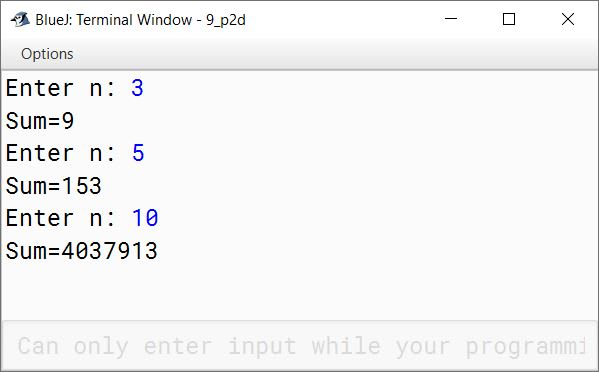(e) S = 1 + (1 + 2) / (1 * 2) + (1 + 2 + 3) / (1 * 2 * 3) + ....... + (1 + 2 + 3 ....... + n) / (1 * 2 * 3 * ....... * n)

```import java.util.Scanner;

public class KboatSeries
{
public void computeSeriesSum() {

Scanner in = new Scanner(System.in);
System.out.print("Enter n: ");
int n = in.nextInt();
double seriesSum = 0.0;
int sum = 0;
double prod = 1.0;

for (int i = 1; i <= n; i++) {
sum += i;
prod *= i;
double term = sum / prod;
seriesSum += term;
}

System.out.println("Sum=" + seriesSum);
}
}```
##### Output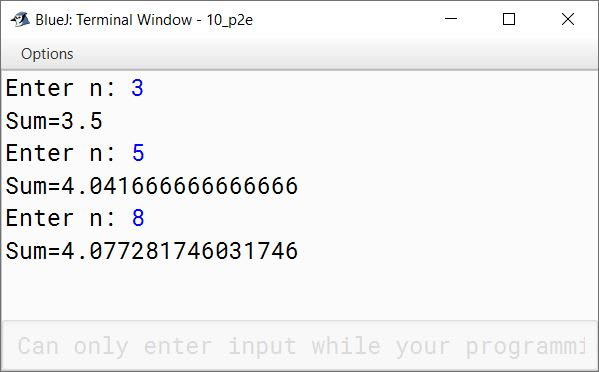#### Question 3

Write the programs to find the sum of the following series:

(a) S = a + a2 + a3 + ....... + an

```import java.util.Scanner;

public class KboatSeries
{
public void computeSeriesSum() {

Scanner in = new Scanner(System.in);
System.out.print("Enter a: ");
int a = in.nextInt();
System.out.print("Enter n: ");
int n = in.nextInt();
int sum = 0;

for (int i = 1; i <= n; i++) {
sum += Math.pow(a, i);
}

System.out.println("Sum=" + sum);
}
}```
##### Output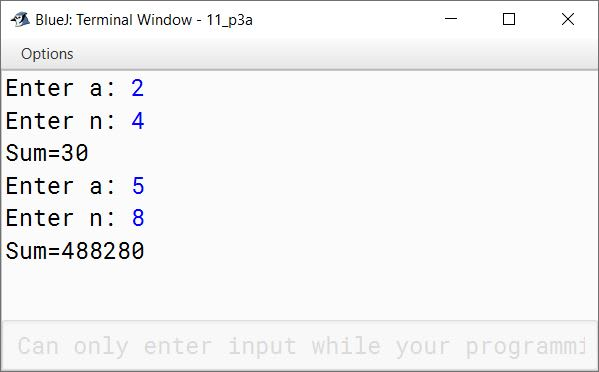(b) S = (a+1) + (a+2) + (a+3) + ....... + (a+n)

```import java.util.Scanner;

public class KboatSeries
{
public void computeSeriesSum() {

Scanner in = new Scanner(System.in);
System.out.print("Enter a: ");
int a = in.nextInt();
System.out.print("Enter n: ");
int n = in.nextInt();
int sum = 0;

for (int i = 1; i <= n; i++) {
sum += a + i;
}

System.out.println("Sum=" + sum);
}
}```
##### Output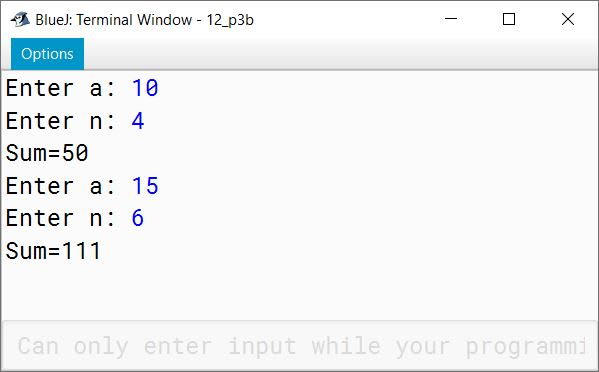(c) S = (a/2) + (a/5) + (a/8) + (a/11) + ....... + (a/20)

```import java.util.Scanner;

public class KboatSeries
{
public void computeSeriesSum() {

Scanner in = new Scanner(System.in);
System.out.print("Enter a: ");
int a = in.nextInt();
double sum = 0;

for (int i = 2; i <= 20; i = i + 3) {
sum += a / (double)i;
}

System.out.println("Sum=" + sum);
}
}```
##### Output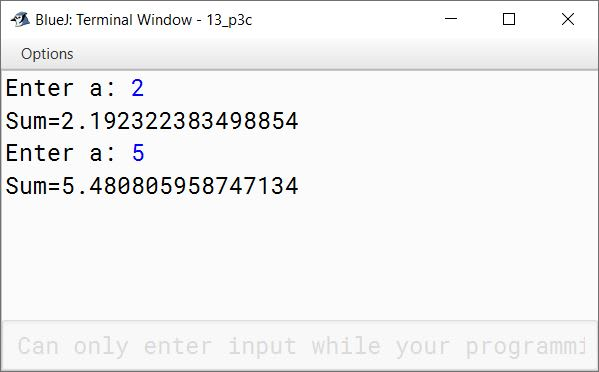(d) S = (1/a) + (2/a2) + (3/a3) + ....... to n

```import java.util.Scanner;

public class KboatSeries
{
public void computeSeriesSum() {

Scanner in = new Scanner(System.in);
System.out.print("Enter a: ");
int a = in.nextInt();
System.out.print("Enter n: ");
int n = in.nextInt();
double sum = 0;

for (int i = 1; i <= n; i++) {
sum += (i / Math.pow(a, i));
}

System.out.println("Sum=" + sum);
}
}```
##### Output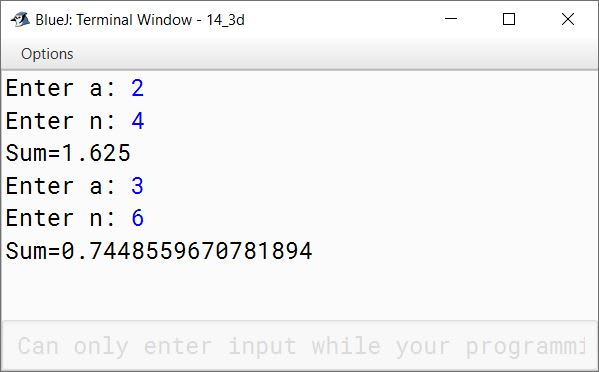(e) S = a - a3 + a5 - a7 + ....... to n

```import java.util.Scanner;

public class KboatSeries
{
public void computeSeriesSum() {

Scanner in = new Scanner(System.in);
System.out.print("Enter a: ");
int a = in.nextInt();
System.out.print("Enter n: ");
int n = in.nextInt();
int sum = 0;

for (int i = 1, j = 1; i <= n; i = i + 2, j++) {
if (j % 2 == 0)
sum -= Math.pow(a, i);
else
sum += Math.pow(a, i);
}

System.out.println("Sum=" + sum);
}
}```
##### Output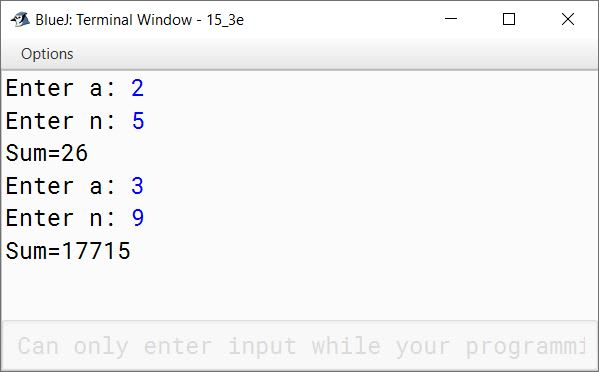#### Question 4

Write a program to enter two numbers and check whether they are co-prime or not.
[Two numbers are said to be co-prime, if their HCF is 1 (one).]
Sample Input: 14, 15
Sample Output: They are co-prime.

```import java.util.Scanner;

public class KboatCoprime
{
public void checkCoprime() {

Scanner in = new Scanner(System.in);
System.out.print("Enter a: ");
int a = in.nextInt();
System.out.print("Enter b: ");
int b = in.nextInt();

int hcf = 1;

for (int i = 1; i <= a && i <= b; i++) {
if (a % i == 0 && b % i == 0)
hcf = i;
}

if (hcf == 1)
System.out.println(a + " and " + b + " are co-prime");
else
System.out.println(a + " and " + b + " are not co-prime");
}
}```
##### Output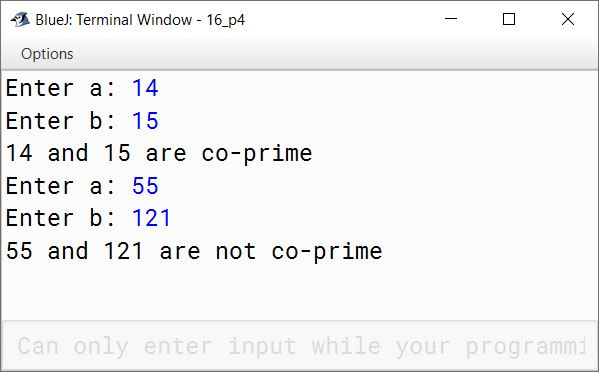#### Question 5

Write a program to input a number. Display the product of the successors of even digits of the number entered by user.
Input: 2745
Output: 15
[Hint: The even digits are: 2 and 4
The product of successor of even digits is: 3*5= 15]

```import java.util.Scanner;

public class KboatEvenSuccessor
{
public void computeProduct() {
Scanner in = new Scanner(System.in);
System.out.print("Enter the number: ");
int num = in.nextInt();
int orgNum = num;
int prod = 1;

while (num != 0) {
int digit = num % 10;
num /= 10;

if (digit % 2 == 0)
prod = prod * (digit + 1);
}

if (prod == 1)
System.out.println("No even digits in " + orgNum);
else
System.out.println("Product of even digits successors is "
+ prod);
}
}```
##### Output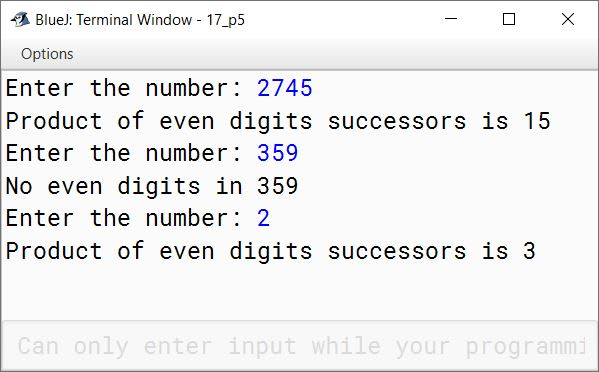#### Question 6

Write a program to input a number and check and print whether it is a Pronic number or not. [Pronic number is the number which is the product of two consecutive integers.]
Examples:
12 = 3 * 4
20 = 4 * 5
42 = 6 * 7

```import java.util.Scanner;

public class KboatPronicNumber
{
public void pronicCheck() {
Scanner in = new Scanner(System.in);
System.out.print("Enter the number to check: ");
int num = in.nextInt();

boolean isPronic = false;

for (int i = 1; i <= num - 1; i++) {
if (i * (i + 1) == num) {
isPronic = true;
break;
}
}

if (isPronic)
System.out.println(num + " is a pronic number");
else
System.out.println(num + " is not a pronic number");
}
}```
##### Output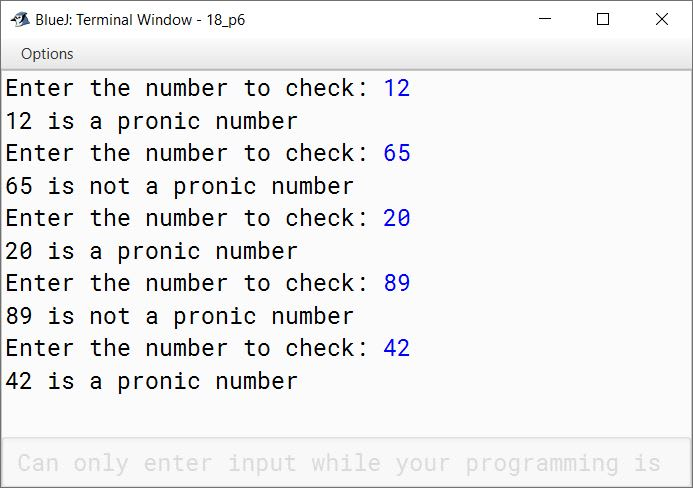#### Question 7

A prime number is said to be 'Twisted Prime', if the new number obtained after reversing the digits is also a prime number. Write a program to accept a number and check whether the number is 'Twisted Prime' or not.
Sample Input: 167
Sample Output: 761
167 is a 'Twisted Prime'.

```import java.util.Scanner;

public class KboatTwistedPrime
{
public void twistedPrimeCheck() {
Scanner in = new Scanner(System.in);
System.out.print("Enter number: ");
int num = in.nextInt();

if (num == 1) {
System.out.println(num + " is not a twisted prime number");
}
else {
boolean isPrime = true;
for (int i = 2; i <= num / 2; i++) {
if (num % i == 0) {
isPrime = false;
break;
}
}

if (isPrime) {

int t = num;
int revNum = 0;

while (t != 0) {
int digit = t % 10;
t /= 10;
revNum = revNum * 10 + digit;
}

for (int i = 2; i <= revNum / 2; i++) {
if (revNum % i == 0) {
isPrime = false;
break;
}
}
}

if (isPrime)
System.out.println(num + " is a twisted prime number");
else
System.out.println(num + " is not a twisted prime number");
}

}
}```
##### Output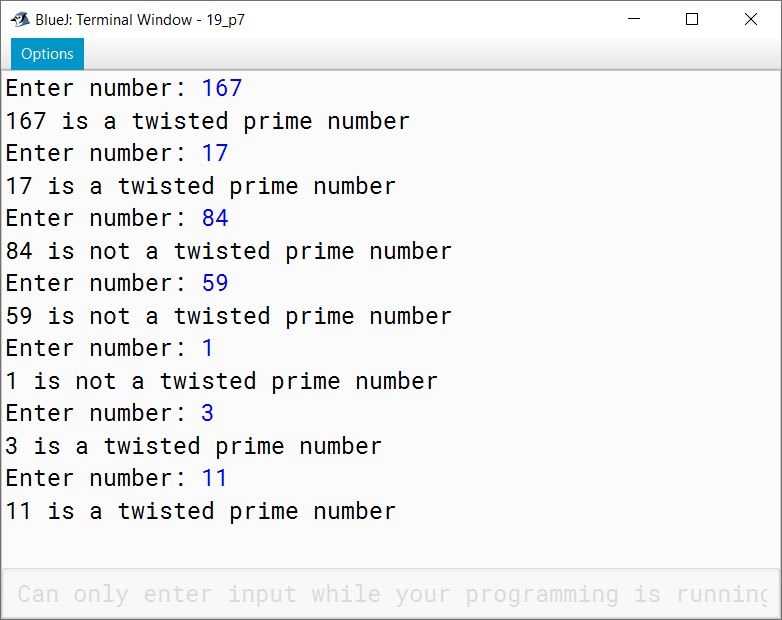#### Question 8

Write a program to input a number and check whether it is a prime number or not. If it is not a prime number then display the next number that is prime.
Sample Input: 14
Sample Output: 17

```import java.util.Scanner;

public class KboatPrimeCheck
{
public void primeCheck() {
Scanner in = new Scanner(System.in);
System.out.print("Enter number: ");
int num = in.nextInt();

boolean isPrime = true;
for (int i = 2; i <= num / 2; i++) {
if (num % i == 0) {
isPrime = false;
break;
}
}

if (isPrime) {
System.out.println(num + " is a prime number");
}
else {
for (int newNum = num + 1; newNum <= Integer.MAX_VALUE; newNum++) {
isPrime = true;
for (int i = 2; i <= newNum / 2; i++) {
if (newNum % i == 0) {
isPrime = false;
break;
}
}
if (isPrime) {
System.out.println("Next prime number = " + newNum);
break;
}
}
}
}
}```
##### Output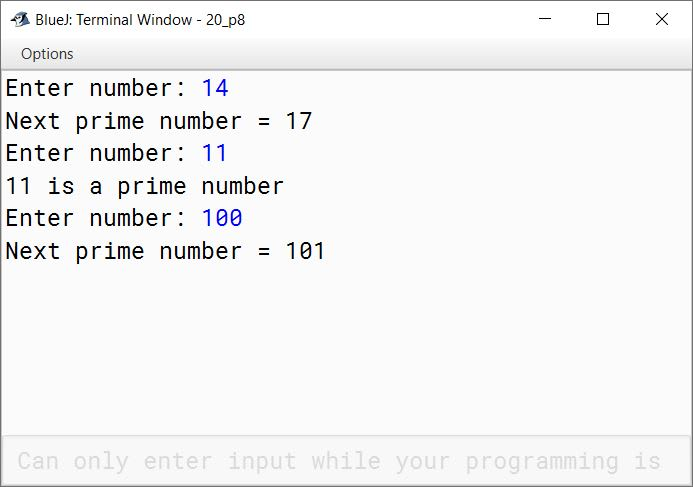#### Question 9

A number is said to be Duck if the digit zero is (0) present in it. Write a program to accept a number and check whether the number is Duck or not. The program displays the message accordingly. (The number must not begin with zero)
Sample Input: 5063
Sample Output: It is a Duck number.
Sample Input: 7453
Sample Output: It is not a Duck number.

```import java.io.BufferedReader;
import java.io.IOException;

public class KboatDuckNumber
{
public void duckNumberCheck() throws IOException {

System.out.print("Enter number: ");
boolean isDuck = false;
boolean firstZero = false;
int c = 0, d;

/*
* 10 is the ASCII code of newline
* We will read from inputstream
* one character at a time till we
* encounter a newline i.e. enter
*/
while((d = in.read()) != 10) {

char ch = (char)d;

if (c == 0 && ch == '0'  )
firstZero = true;

if (!firstZero && ch == '0')
isDuck = true;

c++;
}

if (isDuck)
System.out.println("Duck Number");
else
System.out.println("Not a Duck Number");
}
}```
##### Output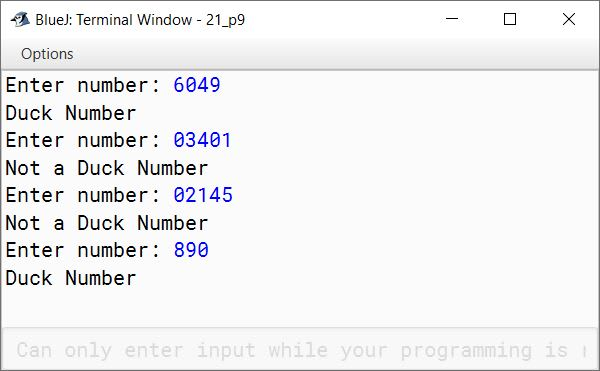#### Question 10

Write a program to input a number and display it into its Binary equivalent.
Sample Input: (21)10
Sample Output: (10101)2

```import java.util.*;

public class KboatDecimaltoBinary
{
public static void main(String args[])  {
Scanner in = new Scanner(System.in);
System.out.print("Enter the decimal number: ");
long decimal = in.nextLong();
long binary = 0, m = 1, rem = 0;

while(decimal > 0)    {
rem = decimal % 2;
binary = binary + (rem * m);
m *= 10;
decimal /= 2;
}
System.out.println("Equivalent binary number: " + binary);
}
}```
##### Output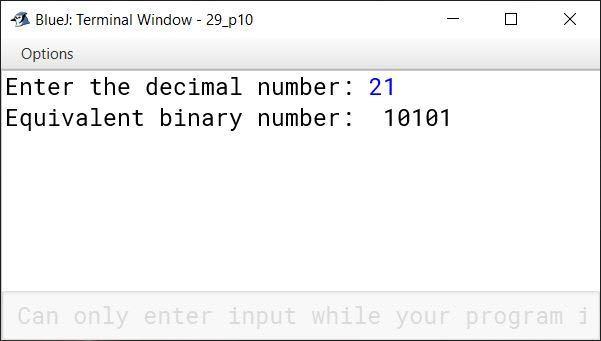#### Question 11

A computerised bus charges fare from each of its passengers based on the distance travelled as per the tariff given below:

Distance (in km)Charges
First 5 km₹80
Next 10 km₹10/km
More than 15 km₹8/km

As the passenger enters the bus, the computer prompts 'Enter distance you intend to travel'. On entering the distance, it prints his ticket and the control goes back for the next passenger. At the end of journey, the computer prints the following:

1. the number of passenger travelled

Write a program to perform the above task.
[Hint: Perform the task based on user controlled loop]

```import java.util.Scanner;

public class KboatBusTravel
{
public void busTravel() {

Scanner in = new Scanner(System.in);
int dist, tf = 0, tp = 0;
System.out.println("Enter distance as -1 to complete the journey");
while (true) {
System.out.print("Enter distance you intend to travel: ");
dist = in.nextInt();
int f = 0;
if (dist == -1) {
break;
}
else if (dist <= 5) {
f = 80;
}
else if (dist <= 15) {
f = 80 + ((dist - 5) * 10);
}
else {
f = 80 + 100 + ((dist - 15) * 8);
}

tf += f;
tp++;

System.out.println("Your fare is " + f);
}

System.out.println("Total Passengers: " + tp);
System.out.println("Total Fare: " + tf);
}
}```
##### Output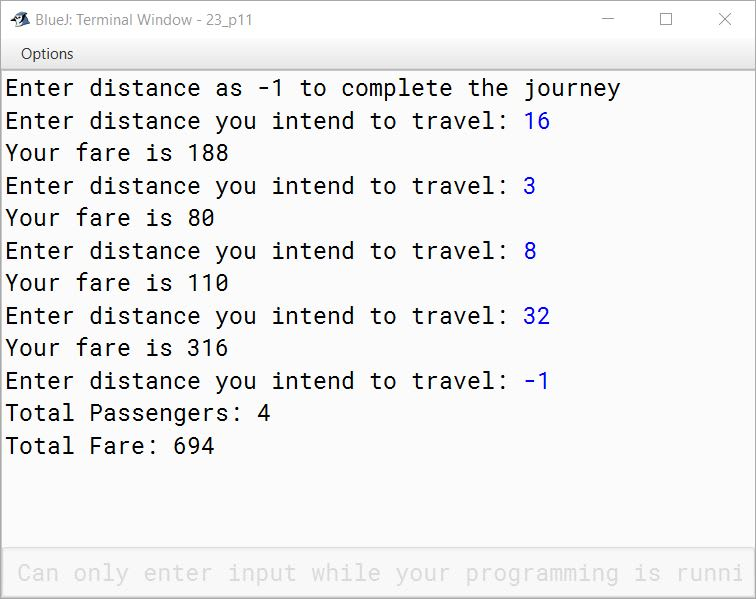#### Question 12

A special two-digit number is such that when the sum of its digits is added to the product of its digits, the result is equal to the original two-digit number.

Example: Consider the number 59.
Sum of digits = 5 + 9 = 14
Product of digits = 5 * 9 = 45
Sum of the sum of digits and product of digits = 14 + 45 = 59

Write a program to accept a two-digit number. Add the sum of its digits to the product of its digits. If the value is equal to the number input, then display the message "Special two—digit number" otherwise, display the message "Not a special two-digit number".

```import java.util.Scanner;

public class KboatSpecialNumber
{
public void checkNumber() {

Scanner in = new Scanner(System.in);

System.out.print("Enter a 2 digit number: ");
int orgNum = in.nextInt();

int num = orgNum;
int count = 0, digitSum = 0, digitProduct = 1;

while (num != 0) {
int digit = num % 10;
num /= 10;
digitSum += digit;
digitProduct *= digit;
count++;
}

if (count != 2)
System.out.println("Invalid input, please enter a 2-digit number");
else if ((digitSum + digitProduct) == orgNum)
System.out.println("Special 2-digit number");
else
System.out.println("Not a special 2-digit number");

}
}```
##### Output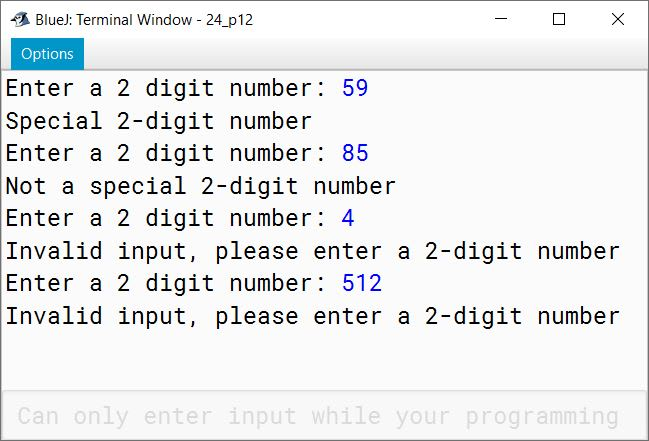#### Question 13

An abundant number is a number for which the sum of its proper divisors (excluding the number itself) is greater than the original number. Write a program to input number and check whether it is an abundant number or not.
Sample input: 12
Sample output: It is an abundant number.
Explanation: Its proper divisors are 1, 2, 3, 4 and 6
Sum = 1 + 2 + 3 + 4 + 6 = 16
Hence, 12 is an abundant number.

```import java.util.Scanner;

public class KboatAbundantNumber
{
public static void main(String args[]) {
Scanner in = new Scanner(System.in);
System.out.print("Enter the number: ");
int n = in.nextInt();
int sum = 0;

for (int i = 1; i < n; i++) {
if (n % i == 0)
sum += i;
}

if (sum > n)
System.out.println(n + " is an abundant number.");
else
System.out.println(n + " is not an abundant number.");
}
}```
##### Output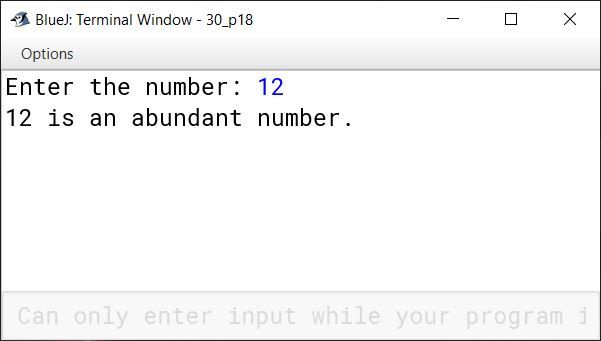#### Question 14

Write a program to accept a number and check whether it is a 'Spy Number' or not. (A number is spy if the sum of its digits equals the product of its digits.)

Example: Sample Input: 1124
Sum of the digits = 1 + 1 + 2 + 4 = 8
Product of the digits = 1*1*2*4 = 8

```import java.util.Scanner;

public class KboatSpyNumber
{
public void spyNumCheck() {

Scanner in = new Scanner(System.in);

System.out.print("Enter Number: ");
int num = in.nextInt();

int digit, sum = 0;
int orgNum = num;
int prod = 1;

while (num > 0) {
digit = num % 10;

sum += digit;
prod *= digit;
num /= 10;
}

if (sum == prod)
System.out.println(orgNum + " is Spy Number");
else
System.out.println(orgNum + " is not Spy Number");

}
}```
##### Output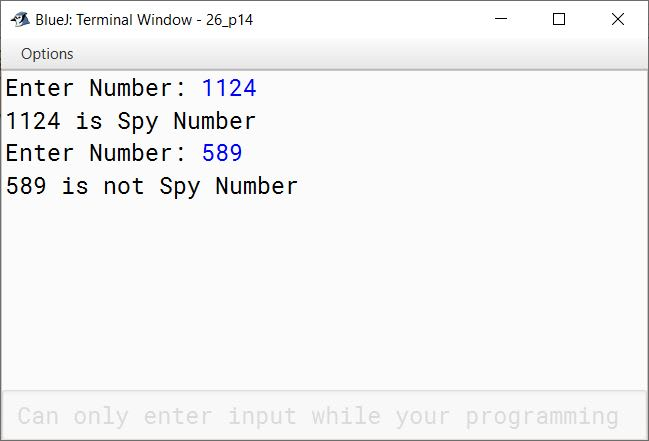#### Question 15

Write a program to input a number and check whether it is a Harshad Number or not. [A number is said to be Harshad number, if it is divisible by the sum of its digits. The program displays the message accordingly.]
For example;
Sample Input: 132
Sum of digits = 6 and 132 is divisible by 6.
Output: It is a Harshad Number.
Sample Input: 353
Output: It is not a Harshad Number.

```import java.util.*;

{
public static void main(String args[])
{
Scanner in = new Scanner(System.in);
System.out.print("Enter a number: ");
int num = in.nextInt();
int x = num, rem = 0, sum = 0;

while(x > 0) {
rem = x % 10;
sum = sum + rem;
x = x / 10;
}

int r = num % sum;

if(r == 0)
System.out.println(num + " is a Harshad Number.");
else
System.out.println(num + " is not a Harshad Number.");
}
}```
##### Output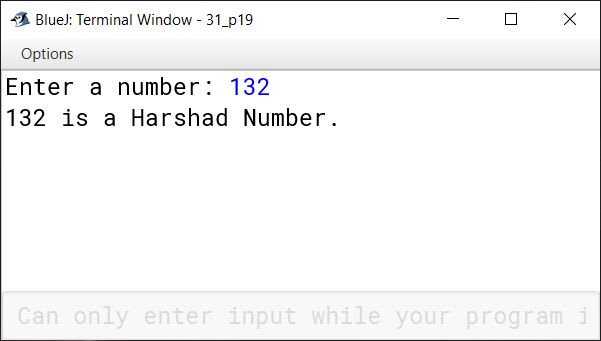#### Question 16

Write a program to display all Pronic numbers in the range from 1 to n.
Hint: A Pronic number is a number which is the product of two consecutive numbers in the form of n * (n + 1).
For example;
2, 6, 12, 20, 30,...........are Pronic numbers.

```import java.util.Scanner;

public class KboatPronicNumber
{
public static void main(String args[])  {
Scanner in = new Scanner(System.in);
System.out.print("Enter the number: ");
int n = in.nextInt();

System.out.println("Pronic numbers from 1 to " + n + " :");

for (int i = 1; i <= n; i++) {
for(int j = 1; j <= i-1; j++)   {
if (j * (j + 1) == i) {
System.out.print(i + " ");
break;
}
}
}
}
}```
##### Output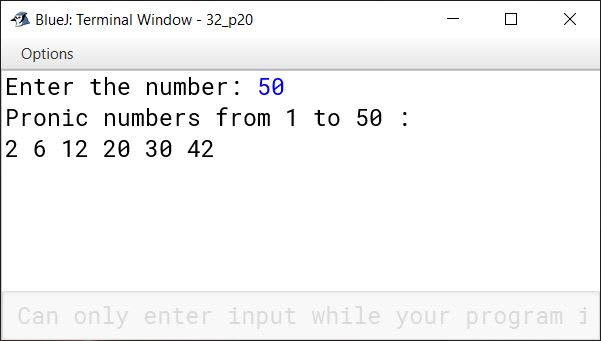#### Question 17

You can multiply two numbers 'm' and 'n' by repeated addition method.
For example, 5 * 3 = 15 can be performed by adding 5 three times ⇒ 5 + 5 + 5 = 15

Similarly, successive subtraction of two numbers produces 'Quotient' and 'Remainder' when a number 'a' is divided by 'b' (a>b).
For example, 5/2 ⇒ Quotient = 2 and Remainder = 1

ProcessResultCounter
5 - 231
3 - 212

Sample Output: The last counter value represents 'Quotient' ⇒ 2
The last result value represents 'Remainder' ⇒ 1

Write a program to accept two numbers. Perform multiplication and division of the numbers as per the process shown above by using switch case statement.

```import java.util.Scanner;

public class KboatArithmetic
{
public void computeResult() {

Scanner in = new Scanner(System.in);
System.out.print("Enter first number: ");
int a = in.nextInt();
System.out.print("Enter second number: ");
int b = in.nextInt();
System.out.println("1. Multiplication");
System.out.println("2. Division");
int ch = in.nextInt();

switch (ch) {
case 1:
int rMul = 0;
for (int i = 1; i <= b; i++) {
rMul += a;
}
System.out.println("Multiplication result = " + rMul);
break;

case 2:
int count = 0, t = a;
while (t > b) {
t -= b;
count++;
}
System.out.println("Divison Result");
System.out.println("Quotient = " + count);
System.out.println("Remainder = " + t);
break;

default:
System.out.println("Incorrect Choice");
break;
}
}

}```
##### Output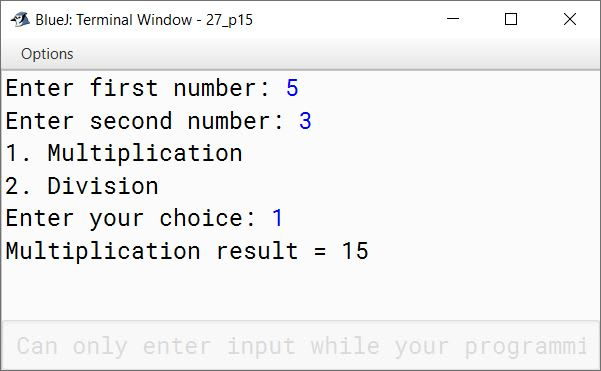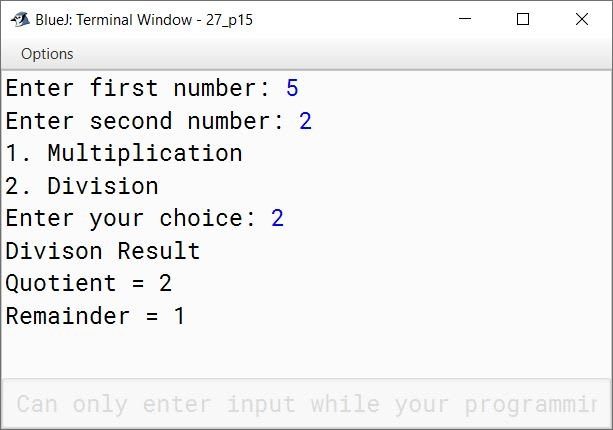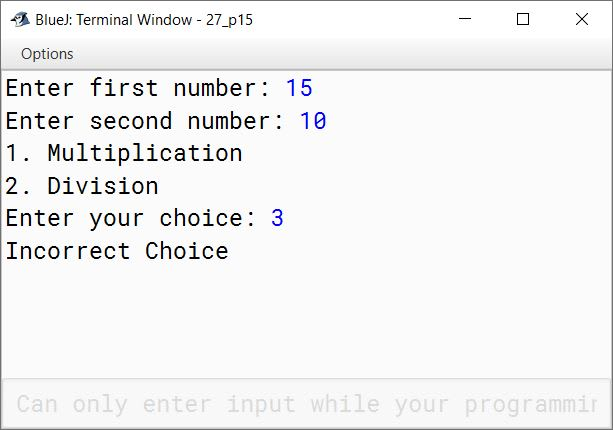#### Question 18

Using a switch statement, write a menu driven program to:

(a) generate and display the first 10 terms of the Fibonacci series
0, 1, 1, 2, 3, 5
The first two Fibonacci numbers are 0 and 1, and each subsequent number is the sum of the previous two.

(b) find the sum of the digits of an integer that is input by the user.
Sample Input: 15390
Sample Output: Sum of the digits = 18
For an incorrect choice, an appropriate error message should be displayed.

```import java.util.Scanner;

public class KboatFibonacciNDigitSum
{
public static void main(String args[]) {
Scanner in = new Scanner(System.in);
System.out.println("1. Fibonacci Series");
System.out.println("2. Sum of digits");
int ch = in.nextInt();

switch (ch) {
case 1:
int a = 0, b = 1;
System.out.print(a + " " + b);
/*
* i is starting from 3 below
* instead of 1 because we have
* already printed 2 terms of
* the series. The for loop will
* print the series from third
* term onwards.
*/
for (int i = 3; i <= 10; i++) {
int term = a + b;
System.out.print(" " + term);
a = b;
b = term;
}
break;

case 2:
System.out.print("Enter number: ");
int num = in.nextInt();
int sum = 0;
while (num != 0) {
sum += num % 10;
num /= 10;
}
System.out.println("Sum of Digits " + " = " + sum);
break;

default:
System.out.println("Incorrect choice");
break;
}
}
}```
##### Output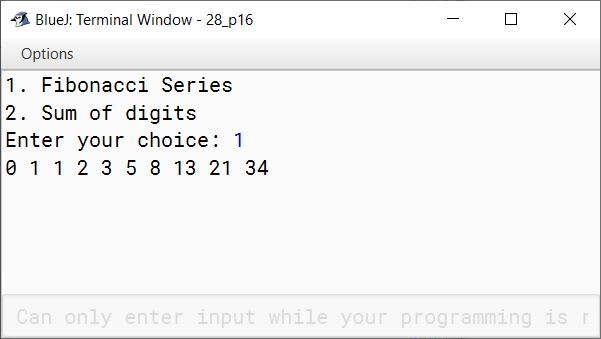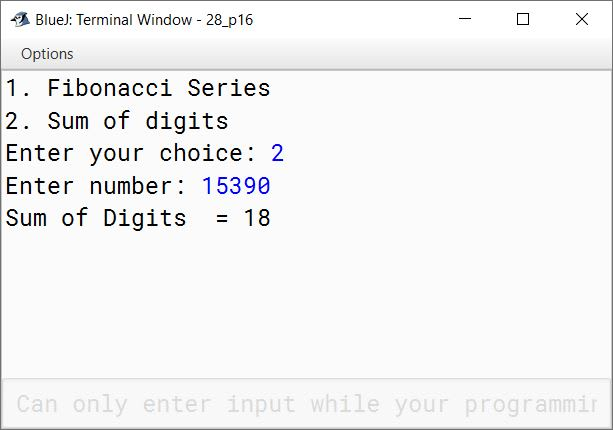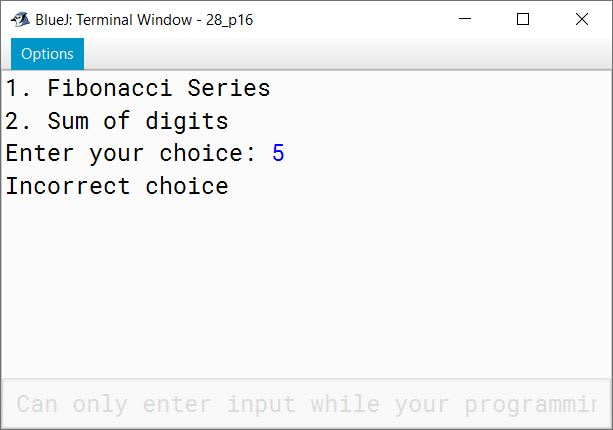#### Question 19

Using switch statement, write a menu driven program for the following:

(a) To find and display the sum of the series given below:

S = x1 - x2 + x3 - x4 + x5 - ....................... - x20

(b) To find and display the sum of the series given below:

S = 1/a2 + 1/a4 + 1/a6 + 1/a8 + ....................... to n terms

For an incorrect option, an appropriate error message should be displayed.

```import java.util.Scanner;

public class KboatSeriesSum
{
public static void main(String args[]) {
Scanner in = new Scanner(System.in);
System.out.println("1. Sum of x^1 - x^2 + .... - x^20");
System.out.println("2. Sum of 1/a^2 + 1/a^4 + .... to n terms");
int ch = in.nextInt();

switch (ch) {
case 1:
System.out.print("Enter the value of x: ");
int x = in.nextInt();
long sum1 = 0;
for (int i = 1; i <= 20; i++) {
int term = (int)Math.pow(x, i);
if (i % 2 == 0)
sum1 -= term;
else
sum1 += term;
}
System.out.println("Sum = " + sum1);
break;

case 2:
System.out.print("Enter the number of terms: ");
int n = in.nextInt();
System.out.print("Enter the value of a: ");
int a = in.nextInt();
int c = 2;
double sum2 = 0;
for(int i = 1; i <= n; i++)  {
sum2 += (1/Math.pow(a, c));
c += 2;
}
System.out.println("Sum = " + sum2);
break;

default:
System.out.println("Incorrect choice");
break;
}
}
}```
##### Output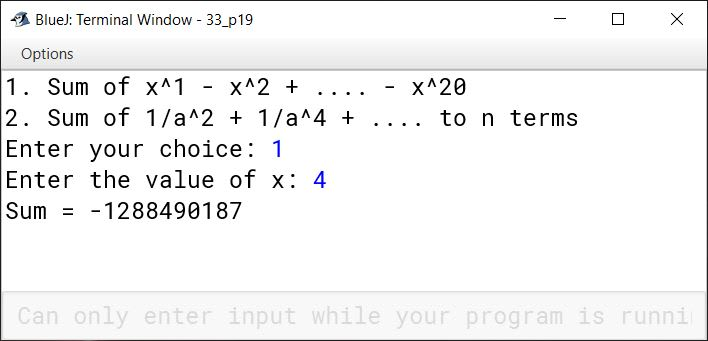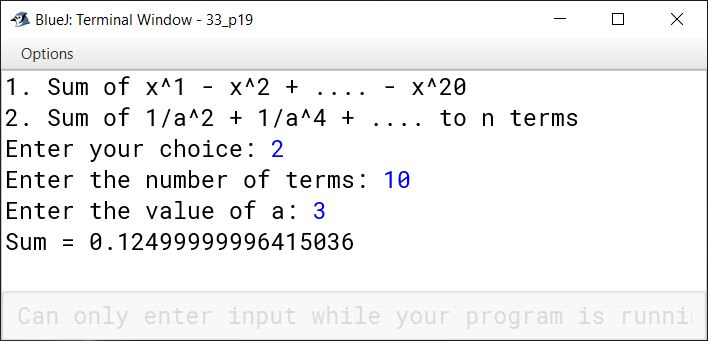#### Question 20

(a) Tribonacci numbers are a sequence of numbers similar to Fibonacci numbers, except that a number is formed by adding the three previous numbers. Write a program to display the first twenty Tribonacci numbers.
For example;
1, 1, 2, 4, 7, 13, ......................

(b) Write a program to display all Sunny numbers in the range from 1 to n.
[Hint: A number is said to be sunny number if square root of (n+1) is an integer.]
For example,
3, 8, 15, 24, 35,......................are sunny numbers.

For an incorrect option, an appropriate error message should be displayed.

```import java.util.Scanner;

public class KboatTribNSunNos
{
public static void main(String args[]) {
Scanner in = new Scanner(System.in);
System.out.println("1. Tribonacci numbers");
System.out.println("2. Sunny numbers");
int ch = in.nextInt();

switch (ch) {
case 1:
int a = 1, b = 1, c = 2;

System.out.print(a + " " + b + " " + c);

for (int i = 4; i <= 20; i++) {
int term = a + b + c;
System.out.print(" " + term);
a = b;
b = c;
c = term;
}
break;

case 2:
System.out.print("Enter n: ");
int n = in.nextInt();

double sqRoot, temp;

for (int i = 3; i <= n; i++)  {
sqRoot = Math.sqrt(i + 1);
temp = sqRoot - Math.floor(sqRoot);
if (temp == 0)
System.out.print(i + " ");
}
break;

default:
System.out.println("Incorrect choice");
break;
}
}
}```
##### Output